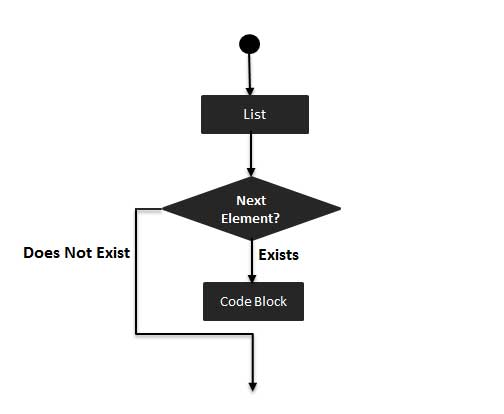# Perl foreach Loop

The foreach loop iterates over a list value and sets the control variable (var) to be each element of the list in turn −

## Syntax

The syntax of a foreach loop in Perl programming language is −

```foreach var (list) {
...
}
```

## Flow Diagram## Example

```#!/usr/local/bin/perl

@list = (2, 20, 30, 40, 50);

# foreach loop execution
foreach \$a (@list) {
print "value of a: \$a\n";
}
```

When the above code is executed, it produces the following result −

```value of a: 2
value of a: 20
value of a: 30
value of a: 40
value of a: 50
```
perl_loops.htm